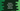# Python Dictionary Built in methods : Python Tutorial 12In our previous tutorial, we have seen how to create a python dictionary and some built-in methods. In this tutorial, we will explore some more dictionary functions, methods and uses.

## 1 .Copy one python Dictionary to another :**

**

To copy a dictionary, we can use copy() method. e.g. :

``````dict1 = { "one" : 1, "two" : 2, "three" : 3, "four" : 4
}

copy_dict = dict1.copy()

print copy_dic``````

The last print statement will print the same values as dict1.

## 2 . Compare Two dictionaries :**

**

For comparing two dictionaries, we can use cmp() method. cmp method takes two parameters as cmp ( dict1, dict2 ) , where dict1 is the first dictionary and dict2 is the second dictionary to be compared . It returns 0 if both dict1 and dict2 are equal. -1 , if dict1 < dict2 and 1 if dict1 > dict2. Let’s try it with and example :

``````dict1 = { "one" : 1, "two" : 2, "three" : 3, "four" : 4
}
dict1_copy = {
"one" : 1, "two" : 2, "three" : 3, "four" : 4
}

dict2 = {
"one" : 1, "two" : 2, "three" : 3, "four" : 5
}

print cmp(dict1, dict1_copy) # 0
print cmp(dict1, dict2) # -1
print cmp(dict2, dict1) # 1``````

## 3 . Get the length of a Dictionary:**

**

To get the size or length of a Dictionary, len() method is used.

``````dict1 = { "one" : 1, "two" : 2, "three" : 3, "four" : 4
}

print len(dict1) # 4``````

## 4. items() method :**

**

items() method returns a list of (key , value) tuple pairs for a dictionary.

``````dict1 = { "one" : 1, "two" : 2, "three" : 3, "four" : 4
}

print dict1.items() # [('four', 4), ('three', 3), ('two', 2), ('one', 1)]``````

## 5 . Get list of python Dictionary keys using keys() method :**

**

``````dict1 = { "one" : 1, "two" : 2, "three" : 3, "four" : 4
}

print dict1.keys() # ['four', 'three', 'two', 'one’]``````

## 6 . Check if a key is exist in a Python dictionary using has_key() method :**

**

``````dict1 = { "one" : 1, "two" : 2, "three" : 3, "four" : 4
}

print dict1.has_key("one") # True
print dict1.has_key("five") # False``````

## 7 . Get value for a key using get() method:

get() method can take one or two parameters. like get(param1) or get(param1 , param2) . param2 is optional and param1 should be the key. If the key is found in the dictionary, it will return the value for that key. If the key is not found, it will return “None” . param2 is the default value that should be returned if the key is not found.

``````dict1 = { "one" : 1, "two" : 2, "three" : 3, "four" : 4
}

print dict1.get("one") # 1
print dict1.get("five") # None
print dict1.get("five",-1) # -1``````

Second and third print statements of the above example are same. But as you can see, if we are passing a default value, it is returned instead of “None” if key is not available .

## 8 . setdefault(key, value ) method :

setdefault() is similar to get() method we have seen above. In the case of get(), if key is not found, the second parameter value is returned. But for setdefault(), if the key is not found, one new item will be created using (key, value) pair and add it to the dictionary. If  key is available, then it will return the value.

``````dict1 = { "one" : 1, "two" : 2, "three" : 3, "four" : 4
}

print dict1.setdefault("one",2) # 1

print dict1 # {'four': 4, 'three': 3, 'two': 2, 'one': 1}

print dict1.setdefault("five",5) # 5

print dict1 # {'four': 4, 'three': 3, 'five': 5, 'two': 2, 'one': 1}``````

## 9 . Get all values of a dictionary:

we can use values() method to get all values from a dictionary.

``````dict1 = { "one" : 1, "two" : 2, "three" : 3, "four" : 4
}

print dict1.values() # [4, 3, 2, 1]``````

## 10 . Convert a dictionary to string :

We can convert a python dictionary to a printable string format :

``````dict1 = { "one" : 1, "two" : 2, "three" : 3, "four" : 4
}

print "Dictionary : %s" %str(dict1) # Dictionary : {'four': 4, 'three': 3, 'two': 2, 'one': 1}``````

using str() method, the dictionary dict1 is converted to a string.